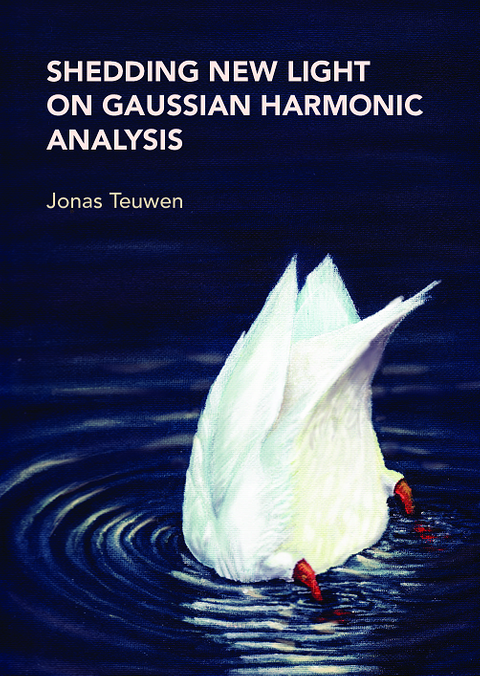# Shedding new light on gaussian harmonic analysis## J. Teuwen

• Promotor: J. van Neerven and P. Urbach
• Graduation year: 2016
• Delft University of Technology

## Abstract

This dissertation consists out of two parts. One part concerns some results on Gaussian harmonic analysis and the other on an optimization problem in optics. In the first part we study the Ornstein-Uhlenbeck process with respect to the Gaussian measure. We focus on two areas. One is on "Gaussian? analogues of classical results in harmonic analysis, and in the second area we study the higher time-derivatives of the integral kernels associated to the Ornstein-Uhlenbeck operator. After introducing the necessary preliminaries on Hermite polynomials, we look at the non-tangential maximal function for the Ornstein-Uhlenbeck semigroup and we prove analogues to classical results. An important distinction here with the classical case is that the maximal function result for the Laplacian allows for t > 0, whereas our result only holds for certain 0 < t < 1 where the exact range depends on the position in space. Next, we compute an explicit formula for the higher time-derivatives of the integral kernel related to the Ornstein-Uhlenbeck operator. As an application we show several kernel bounds using our formula. Finally, as far as the mathematical part is concerned, we study off-diagonal estimates related to the Ornstein-Uhlenbeck operator. It is well-known that these hold for the Laplacian with respect to the Lebesgue measure, but do these hold for the Ornstein-Uhlenbeck operator with respect to the Gaussian measure? It is known that we always would have L2-L2 bounds, even for all t > 0, but in applications one often wants L2-L1 bounds. Even though these do hold for the Laplacian, we show that these cannot hold for the Ornstein-Uhlenbeck operator even for small 0 < t < 1. Moreover, our proof shows that letting the maximal t depend on the position in space will not work either. In the final part of this dissertation we study a problem in theoretical optics. Here we study the optimization of the electric field induced by light as a plane wave in a disk with given radius. We study the electric fields in the lens pupil and the focal region for several radii in the orde of magnitude of the wave length of the light used.